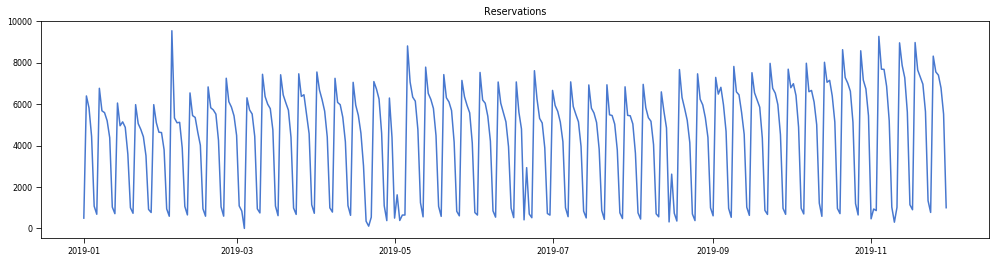# Detecting anomalies in a reservation system using STLDaily reservations

In order to monitor the correct operation of an online reservation system, we have decided to focus on the number of daily reservations. In this way, if we detect that the amount varies significantly away from the trend that the previous days and weeks followed, we could be in the presence of a bug in our system.

To detect these outliers, we use a decomposition algorithm known as STL.

# STL decomposition

STL is a robust method to decompose series into three main components: trend, seasonality, noise. STL is an acronym for “Seasonal and Trend decomposition using Loess”.

• Trend: The increasing or decreasing value in the series.
• Seasonality: The repeating short-term cycle in the series.
• Noise: The random variation in the series.

There are two ways to decompose using STL:

• Additive: y = t + s + r
• Multiplicative: y = t * s * r

In our case, the series behaves seasonally with weekly cycles and has a slightly increasing trend, so an additive decomposition would be the most appropriate option.

If we look closely at the noise we can see that there are points where there is a considerable deviation from the rest. This means that trend and seasonality are not good enough to approximate the function. In this case, they could be outliers.

First we must check that our residuals follow approximately a normal distribution. If it doesn’t then the anomalies may be precisely due to the non-normality of the data. For this we will use a Normal Probability Plot, which is a visual way of checking that a set of data behaves approximately like a normal distribution.

We can see that they approach a straight line except at the extremes where they are far from normal. This indicates that the residual follows a normal distribution and that the extremes are deviations.

We could also perform a normality test if we wanted to be more rigorous.

How to know then which residuals are significantly deviated from the mean. For this we can use some statistical methods to calculate dispersion and variability. Such as variance, standard deviation, average absolute deviation AAD, median absolute deviation MAD.

In our case we have used the median absolute deviation (MAD) because it is a robust method to calculate the variability

Knowing the median absolute deviation and setting a threshold we can assume that everything below or above this value is an anomaly.

# Conclusion

As we have seen before using TLS and MAD we can obtain a simple method to detect anomalies. Unfortunately this method is not valid if the series are not seasonal or when the series changes dramatically.

Software Developer at Docplanner

## More from Alejandro Dominguez

Software Developer at Docplanner